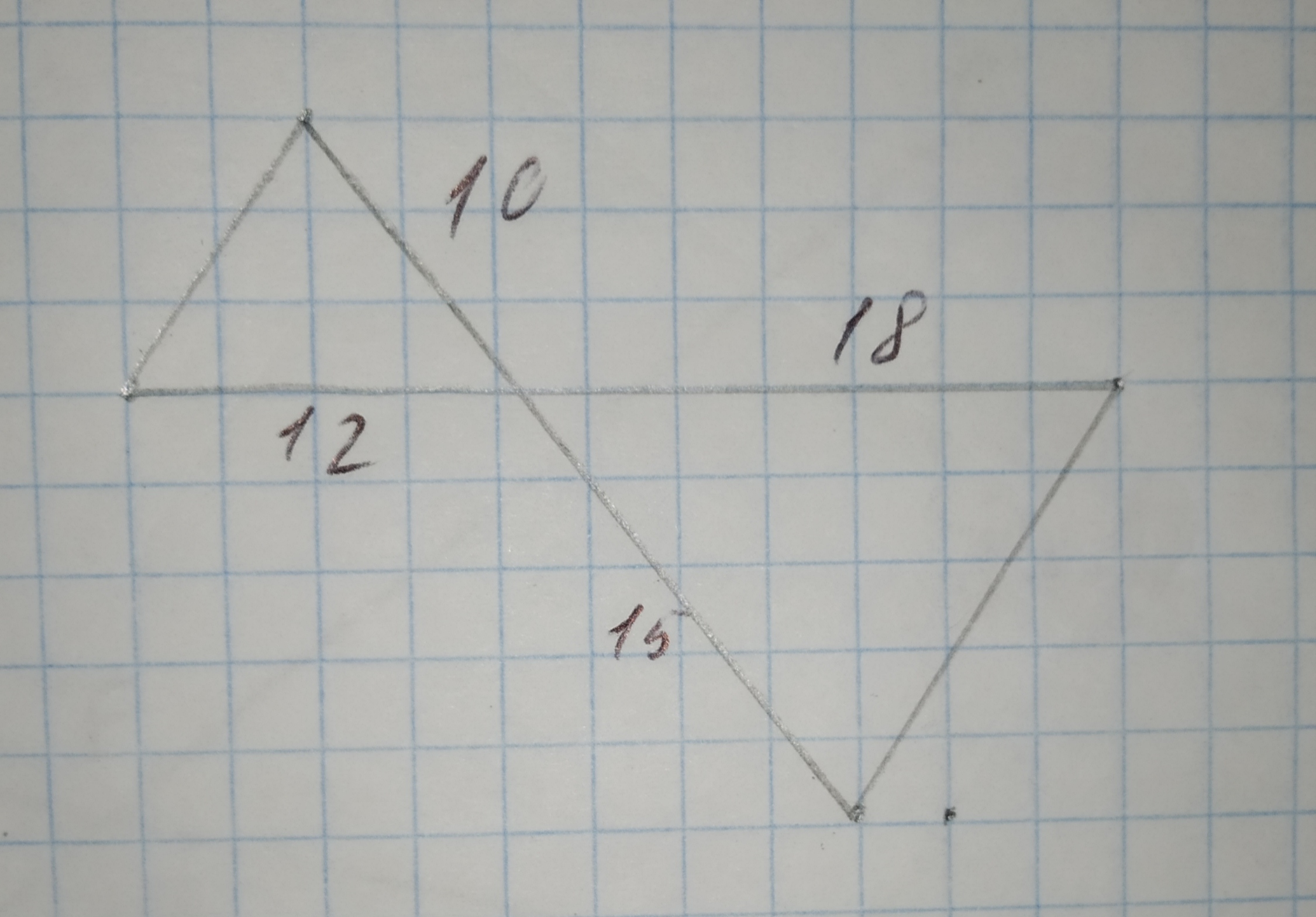Question# To prove:The two triangles are similar using postulate or theorem.

Similarity
ANSWEREDTo prove:The two triangles are similar using postulate or theorem.
Given information:
The system triangles:2021-01-20
Calculation:
Consider two triangles WXV and ZXY as shown as in the textbook.
$$\displaystyle\angle{W}{X}{V}\stackrel{\sim}{=}\angle{Z}{X}{Y}$$
They are vertically opposite angles
Compare the corresponding sides, $$\displaystyle\frac{{{W}{X}}}{{{Z}{X}}}=\frac{{10}}{{15}}=\frac{{2}}{{3}}$$
Compare the corresponding sides, $$\displaystyle\frac{{{V}{X}}}{{{Y}{X}}}=\frac{{12}}{{18}}=\frac{{2}}{{3}}$$
Thus,
$$\displaystyle\frac{{{W}{X}}}{{{Z}{X}}}=\frac{{{V}{X}}}{{{Y}{X}}}$$
$$\displaystyle\frac{{2}}{{3}}=\frac{{2}}{{3}}$$
According to the SAS similarity theorem:
Therefore,
$$\displaystyle\triangle{W}{X}{V}\sim\triangle{Z}{X}{Y}$$
Hence, $$\displaystyle\triangle{W}{X}{V}\sim\triangle{Z}{X}{Y}$$ are similar with each other by the SAS similarity theorem